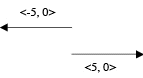# Vectors

Author: Mark Szymczyk
Last Update: August 20, 2005

Vectors are important in game development, especially in the areas of physics and 3D graphics. You can use vectors to represent a game object’s position in the game world, its velocity, and its acceleration. Vectors are also used to respond to collisions between objects. 3D graphics uses vectors for numerous operations, including lighting calculations, bump mapping, and environment mapping. This article introduces you to vectors and vector math.

## Introduction

Before defining what a vector is, I need to define what a scalar quantity is. A scalar quantity has magnitude only. Examples of scalar quantities are 51, 1.34, and –12.56. A vector quantity has both magnitude and direction. Traveling 35 miles per hour on a motorcycle is a scalar quantity. Traveling 35 miles per hour north is a vector quantity.

Vector quantities use the sign to signify direction. The vector quantities 5 and –5 are the same vector with opposite directions, which you can see in Figure 1.

The most common vectors used in computer programs are 2D and 3D vectors. 2D vectors have two components: x and y. 3D vectors have three components: x, y, and z. I’m going to use 3D vectors for the examples in this article. If you can understand 3D vectors, you’ll have no trouble with 2D vectors.Figure 1: Two vectors with the same magnitude, but different directions.

Next (Vector Length)